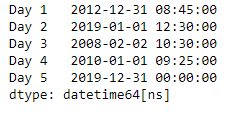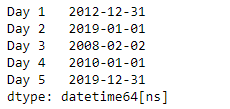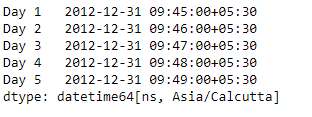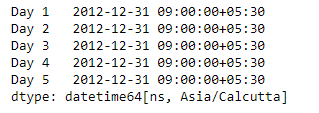# Python | Pandas Series.dt.floor

• Last Updated : 20 Mar, 2019

`Series.dt` can be used to access the values of the series as datetimelike and return several properties. Pandas` Series.dt.floor()` function perform floor operation on the data to the specified freq.

Syntax: Series.dt.floor(*args, **kwargs)

Attention geek! Strengthen your foundations with the Python Programming Foundation Course and learn the basics.

To begin with, your interview preparations Enhance your Data Structures concepts with the Python DS Course. And to begin with your Machine Learning Journey, join the Machine Learning - Basic Level Course

Parameter :
freq : The frequency level to floor the index to

Returns : DatetimeIndex, TimedeltaIndex, or Series

Example #1: Use `Series.dt.floor()` function to floor the datetime data of the given series object to the specified frequency.

 `# importing pandas as pd``import` `pandas as pd`` ` `# Creating the Series``sr ``=` `pd.Series([``'2012-12-31 08:45'``, ``'2019-1-1 12:30'``, ``'2008-02-2 10:30'``,``               ``'2010-1-1 09:25'``, ``'2019-12-31 00:00'``])`` ` `# Creating the index``idx ``=` `[``'Day 1'``, ``'Day 2'``, ``'Day 3'``, ``'Day 4'``, ``'Day 5'``]`` ` `# set the index``sr.index ``=` `idx`` ` `# Convert the underlying data to datetime ``sr ``=` `pd.to_datetime(sr)`` ` `# Print the series``print``(sr)`

Output :Now we will use `Series.dt.floor()` function to floor the datetime values in the given series object to Daily frequency.

 `# floor to daily frequency``result ``=` `sr.dt.floor(freq ``=` `'D'``)`` ` `# print the result``print``(result)`

Output :As we can see in the output, the `Series.dt.floor()` function has successfully floored the datetime values in the given series object to the specified frequency.

Example #2 : Use `Series.dt.floor()` function to floor the datetime data of the given series object to the specified frequency.

 `# importing pandas as pd``import` `pandas as pd`` ` `# Creating the Series``sr ``=` `pd.Series(pd.date_range(``'2012-12-31 09:45'``, periods ``=` `5``, freq ``=` `'T'``,``                            ``tz ``=` `'Asia / Calcutta'``))`` ` `# Creating the index``idx ``=` `[``'Day 1'``, ``'Day 2'``, ``'Day 3'``, ``'Day 4'``, ``'Day 5'``]`` ` `# set the index``sr.index ``=` `idx`` ` `# Print the series``print``(sr)`

Output :Now we will use `Series.dt.floor()` function to floor the datetime values in the given series object to Hourly frequency.

 `# floor to hourly frequency``result ``=` `sr.dt.floor(freq ``=` `'H'``)`` ` `# print the result``print``(result)`

Output :As we can see in the output, the `Series.dt.floor()` function has successfully floored the datetime values in the given series object to the specified frequency.

My Personal Notes arrow_drop_up